# Impulse Physics – Get To Know The BasicsHave you ever hit a punching bag in a gym, kicked a ball in the field, or used a bat in cricket? Well, it means that you have been practicing impulse physics unknowingly. Therefore, what exactly is impulse? In this post, we are going to delve deeper into the concept of impulse physics and its relation to momentum. Finally, we will demonstrate how you can calculate impulse using the impulse formula.

## What is Momentum?

Before digging deeper and answer the question, what is impulse in physics, we will start by looking at momentum. What is momentum?

Momentum is the measure of strength and how hard (difficult) it is to stop an object in motion. It measures how much mass (such as a moving car, ball, or athlete) is in what motion. For example, an object that is in stationary, no matter how heavy it is, has zero momentum. However, a fast-moving small object such as a bullet has a lot of momentum. To get the momentum of an object, we use the formula below: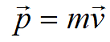(Where M is the mass and V is the velocity)

From this formula, momentum is calculated by multiplying mass and velocity. Therefore, it is a vector quantity. The formula also indicates that if you double either the mass or velocity, you will double the momentum of the object under consideration.

### Momentum and Impulse: How are they Related?

Now that you know what momentum is, we will move on to demonstrate its relation to impulse with some impulse calculations. The concept of impulse physics is based on Newton’s Second Law of motion that states, ‘the acceleration of an object is directly proportional to the net force acting upon the object and inversely proportional to the object’s mass.’

When a force acts, for a short time, on an object, the impact is the measure of the extent that the force changes the object’s momentum. Therefore, from the equation on momentum physics above, the impulse can be represented using the impulse formula below.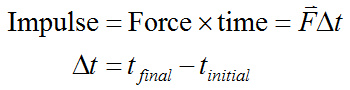Since impulse is the measure of the change in momentum of an object when a force acts on it, an alternative formula for calculating impulse looks like the one provided below: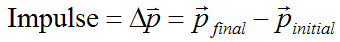Impulse can be presented in two different ways. One, as kilogram times meters per second (kg m/s) or Newton times second (Ns).

When exploring what impulse is, the primary goal is to understand the physics of collision. The main reason why physics impulse is important is that forces are, in most cases, not constant. For example, forces from engines or people build up from zero and could end up varying because of forces working on it.

### Specific Impulse Physics

In momentum and impulse, specific impulse is a quantification that is commonly applied in aerodynamic. It is given to engines that generate thrust force. Good examples include rocket engines and jet engines. Specific impulse is used to measure the efficiency of using specific fuels to generate thrust in such space vehicles.

Note that the use of the prefix specified in this case, just like in specific gravity and specific heat, implies ‘relative’ to a specific quantity. Therefore, specific impulse refers to impulse calculated relative to the jet/space vehicle’s weight of fuel (on earth), used to generate an impulse. See the equation used to calculate specific impulse below: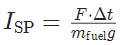From the above equation, you will note that we are dividing the impulse by force (the force on the fuel because of earth’s gravity). Therefore, the force cancels out, which implies that specific impulse in space vehicles is simply given in seconds.

For example, a rocket’s impulse might be 300 s. This implies that the jet could use 1 N fuel to create 1 N of thrust for 300 s.

NOTE: In practice, the jet would have lower thrust (Something like 100 N). This means that the jet would use 1 N fuel to generate 100 N thrust for 3 seconds.

### How to Use the Impulse Formula

How to calculate impulse physics? Now that we have looked at the impulse definition physics, how do you go about calculating it in a real physics assignments problem? Here, we will demonstrate how to calculate impulse using some examples:

Example one: Calculate the impulse of an object that collides with a wall and stops immediately after the collision. The object was 2.0 kilograms and was traveling at a velocity of 10 m/s before hitting the wall. Here is how to calculate the impulse.

Δ p = p f – p i

Δ p = m v f – m v i

Δ p = (2.0 kg) (0 m/s) – (2.0 kg) (10 m/s) = -20 kg m/s

Example two: How do you calculate the impulse of an object that collides with a wall and bounces after the collision? If the object is 2.0 kilograms and is traveling at a velocity of 10 m/s and the speed reduces to -10 m/s, here is how to calculate the impulse.

Note: The velocity after the collision is negative because the object bounces back in the opposite direction).

Δ p = p f – p i

Δ p = m v f – m v i

Δ p = (2.0 kg) (0 m/s) – (2.0 kg) (10 m/s) = -20 kg m/s

Unlike other subjects that are mainly theoretical, impulse physics has applications almost in every area of your life. From hitting a punching bag in the gym to the operation of airbags in motor vehicles, it is all about momentum and impulse. This makes impulse physics definition, associated calculations, and even solving related problems in assignments truly fun. You only need to follow the impulse formulas provided in this post to calculate impulse or demonstrate its relation to moment easily.

Even as we simplify the physics impulse, you should not feel left out if the entire concept and calculations look complex. Well, they can be complex until you understand how to solve them by applying the impulse formula. But before you master the calculations, you need to have the current impulse physics assignment done. The best solution is to seek assistance from homework help services.

Homework help firms provide college students with affordable writing services for impulse physics and other assignments they find tough. If you’re thinking “I need help with my homework,” then this is the fastest solution. We have expert writers who know what impulse physics or other topics are, and how to craft winning papers. Do not let tight deadlines, other engagements, or poor understanding of physics concepts stand on your way to better grades; get help from experts.

No matter how complex your momentum and impulse assignment or question appears, this post will help you to make it simple and enjoyable. No need for stress anymore!

Let's stand with the heroes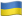As Putin continues killing civilians, bombing kindergartens, and threatening WWIII, Ukraine fights for the world's peaceful future.

Ukraine Live Updateshttps://war.ukraine.ua/
Donate Directly to Ukraine